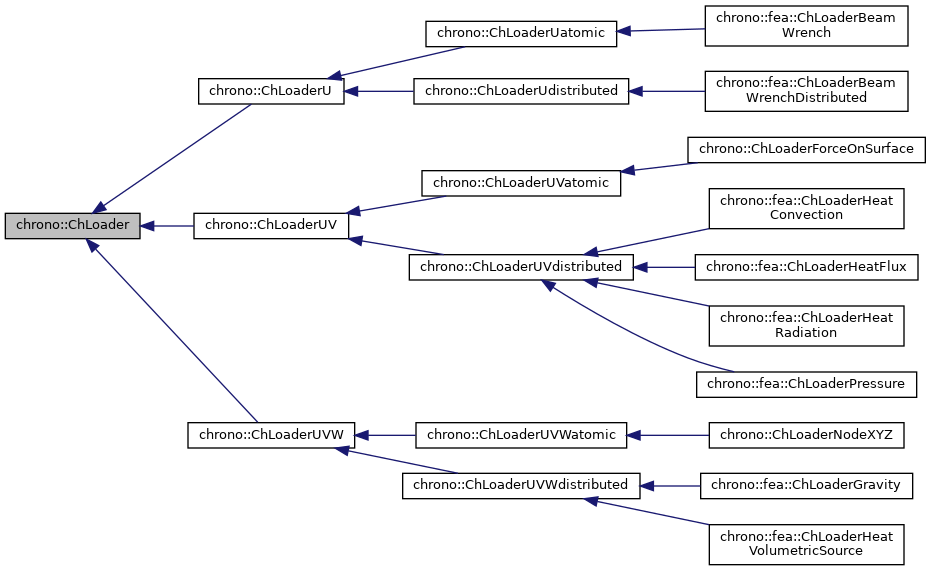Description

Class for loads applied to a single ChLoadable object.

Loads can be forces, torques, pressures, thermal loads, etc. depending on the loaded ChLoadable object. For example if the load references a ChBody, the load is a wrench (force+torque), for a tetrahedron FE it is a force, etc. Objects of this class must be capable of computing the generalized load Q from the load F.

Inheritance diagram for chrono::ChLoader:[legend]

Public Member Functions

virtual void ComputeQ (ChVectorDynamic<> *state_x, ChVectorDynamic<> *state_w)=0

virtual bool IsStiff ()

Public Attributes

ChVectorDynamic Q

Member Function Documentation

 virtual void chrono::ChLoader::ComputeQ ( ChVectorDynamic<> * state_x, ChVectorDynamic<> * state_w )
pure virtual
Parameters
 state_x if != 0, update state (pos. part) to this, then evaluate Q state_w if != 0, update state (speed part) to this, then evaluate Q